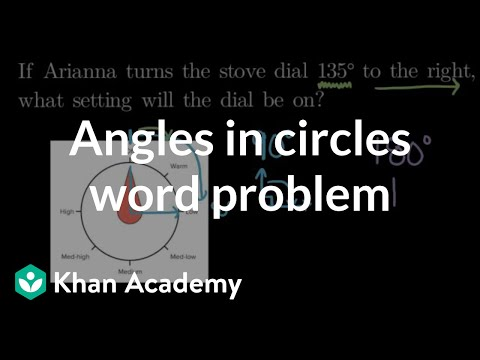Video

# Measuring angles in degrees (Full video)

Description: Learn how to measure an angle with a protractor. Created by Sal Khan. Now that we know what an angle is, let's think about how we can measure them. And we already hinted at one way to think about the measure of angle in the last video where we said, look, this angle XYZ seems more open than angle BAC. So maybe the measure of angle XYZ should be larger than the angle of the BAC, and that is exactly the way we think about the measures of angles.

### Other videos you might be interested in### Angle measurement & circle arcs (Full video)